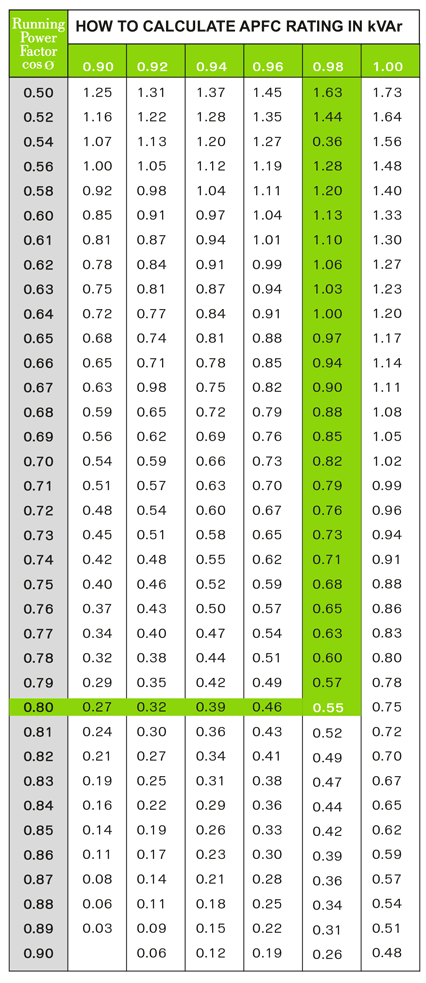Method (1)

Required Panel kVAr:
Example :
Running PF
Desired PF
Multiply Factor From Table
Connected Capacitor + ( KW x Multiply factor )
Required Panel kVAr = 20 + ( 140 x 0.55) = 97

Method (2)

kVAr Required = KW x Month Electricity Bill
Calculation for Electricity Bill = ________
KW per hour = Monthly KWH on Electricity Bill / Working x Working hours
Power Factor Electricity bill = _________
Required kVAr = KW x Multiply Factor = _______ x _______